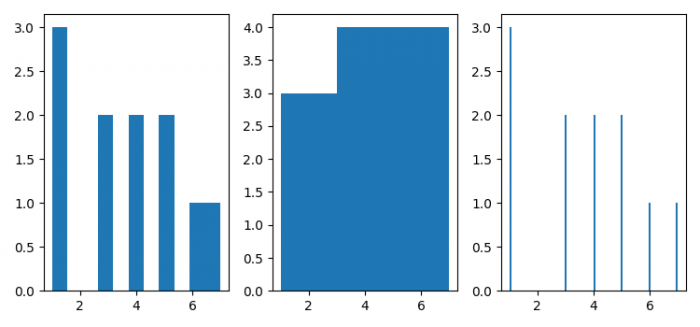# How is the Pyplot histogram bins interpreted? (Matplotlib)

To plot histogram bins interpreted with different bins, we can take the following steps −

• Set the figure size and adjust the padding between and around the subplots.

• Make a list of data to plot in histogram.

• Add a subplot to the current figure,nrows=1, ncols=3 and index=1.

• Plot a histogram with data; bins is a number.

• Add a subplot to the current figure, nrows=1, ncols=3 and index=2.

• Plot a histogram with data; bins is an array.

• Add a subplot to the current figure, nrows=1, ncols=3 and index=3.

• Plot a histogram with data, bins is a string.

## Example

import matplotlib.pyplot as plt

plt.rcParams["figure.figsize"] = [7.50, 3.50]
plt.rcParams["figure.autolayout"] = True

data = [1, 3, 1, 4, 7, 1, 3, 5, 4, 6, 5]

plt.subplot(131)
plt.hist(data, bins=len(data))

plt.subplot(132)
plt.hist(data, bins=[1, 3, 5, 7])

plt.subplot(133)
plt.hist(data, bins='stone')

plt.show()

## Output